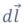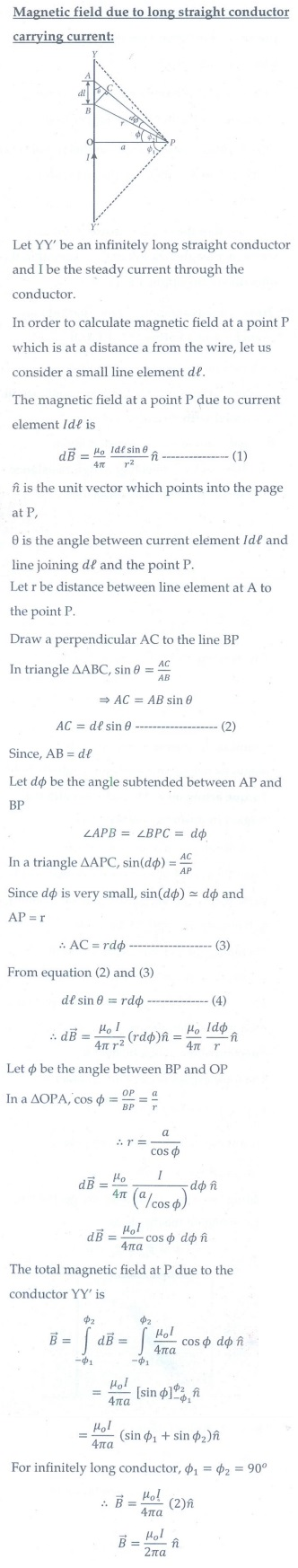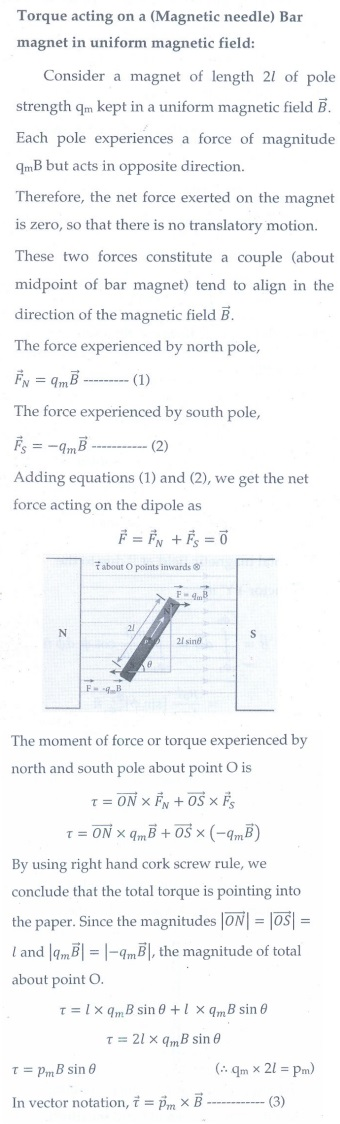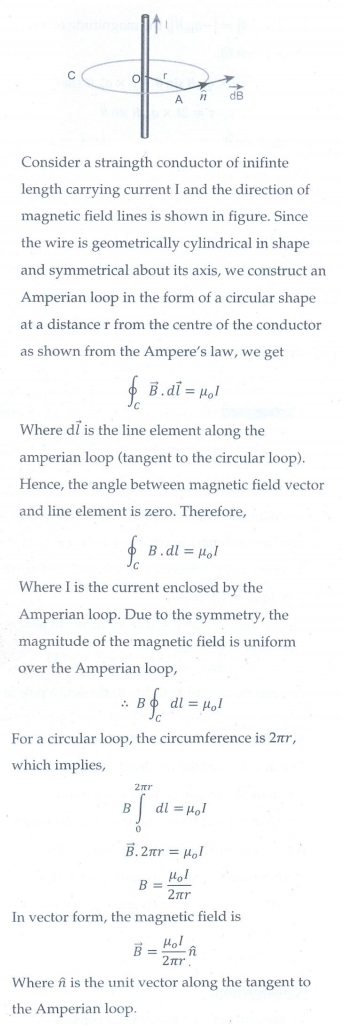Home | | Physics 12th Std | Magnetism and Magnetic Effects of Electric Current: Questions and Answers

# Magnetism and Magnetic Effects of Electric Current: Questions and Answers

Physics : Magnetism and Magnetic Effects of Electric Current: Important Questions, Short answer questions, Long answer questions

1. What is meant by magnetic induction?

The magnetic induction (total magnetic field) inside the specimenis equal to the sum of the magnetic fieldo produced in vacuum due to the magnetizing field and the magnetic fieldm due to the induced magnetism of the substance.2. Define magnetic flux.

The number of magnetic field lines crossing any area normally is defined as magnetic flux ϕB through the area.It is a scalar quantity.

Its SI unit is weber.

Its dimension is [ML2T-2A-1]

3. Define magnetic dipole moment.

The magnetic dipole moment is defined as the product of its pole strength and magnetic length. It is a vector quantity, denoted by.Whereis the vector drawn from south pole to north pole and its magnitude || = 2l.

The magnitude of magnetic dipole moment is Pm = 2qml

SI unit is Am2

4. State Coulomb’s inverse law.

The force of attraction or repulsion between two magnetic poles is directly proportional to the product of their pole strengths and inversely proportional to the square of the distance between them.Where mand mB are pole strengths of two poles and is the distance between two magnetic poles.

5. What is magnetic susceptibility?

It is defined as the ratio of the intensity of magnetization () induced in the material due to the magnetizing field ()It is a dimensionless quantity.

For an isotropic medium, susceptibility is a scalar but for non-isotropic medium, susceptibility is a tensor.

6. State Biot-Savart’s law.

Biot and Savart experimentally observed that the magnitude of magnetic fieldat a point P at a distance r from the small elemental length taken on a conductor carrying current varies.

(i) directly as the strength of the current I

(ii) directly as the magnitude of the length element(iii) directly as the sine of the angle (say, θ) betweenand.

(iv) inversely as the square of the distance between the point P and length element.

This is expressed as7. What is magnetic permeability?

The magnetic permeability can be defined as the measure of ability of the material to allow the passage of magnetic field lines through it or measure of the capacity of the substance to take magnetization or the degree of penetration of magnetic field through the substance.

In free space, the permeability (or absolute permeability) is denoted by µ0 = 4π × 10 -7 Hm-1

8. State Ampere’s circuital law.

Ampere's law: The line integral of magnetic field over a closed loop is μo times net current enclosed by the loop.Where Ienclosed is the net current linked by the closed loop.

9. Compare dia, para and ferro-magnetism.10.    What is meant by hysteresis?

The phenomenon of lagging of magnetic induction behind the magnetizing field is called hysteresis. Hysteresis means ‘lagging behind'.

11. Define magnetic declination and inclination.

Magnetic declination (D):

The angle between magnetic meridian at a point and geographical meridian is called the magnetic declination.

Magnetic inclination (or) dip(I):

The angle subtended by the Earth's total magnetic fieldwith the horizontal direction in the magnetic meridian is called dip or magnetic inclination at that point.

12. What is resonance condition in cyclotron?

In cyclotron operation, the frequency f at which the positive ion circulates in the magnetic field must be equal to the constant frequency of the electrical oscillator fosc. This is called resonance condition in cyclotron.13. Define ampere.

One ampere is defined as that current when it is passed through each of the two infinity long parallel straight conductors kept at a distance of one meter apart in vacuum causes each conductor to experience a force of 2 x 10-7 newton per meter length of conductor.

14. State Fleming's left hand rule.

Stretch forefinger, the middle finger and the thumb of the left hand such that they are in mutually perpendicular directions. If we keep the forefinger in the direction of magnetic field, the middle finger in the direction of the electric current, then the thumb points in the direction of the force experienced by the conductors.

15. Is an ammeter connected in series or parallel in a circuit? Why?

An ammeter connected in series in a circuit.

In an ammeter a shunt resistance is connected in parallel to galvanometer. Since, the shunt reistance is a very low resistance, the resistance of ammeter is also very small. So, when we connect the ammeter in series, the ammeter will not change appreciably the current in the circuit.

16. Explain the concept of velocity selector.

Velocity selector:In the region of space between the parallel plates of a capacitor which produce uniform electric field, a uniform magnetic fieldis maintained perpendicular to the direction of electric field.

Suppose a charged particle with charge q enters the space from left side with a velocity, the net force on the particle isFor a positive charge, the electric force on the charge acts in downward direction where as the Lorentz force acts upwards. When these two forces balance each other, then

qE = qvoB

vo = E/B

This means, for a given magnitude ofand, the forces act only on the particle moving with particular speed v0 =E/B. This speed is independent of mass and charge.

By proper choice of electric and magnetic fields, the particle with particular speed can be selected. Such an arrangement of fields is called a velocity selector.

17. Why is the path of a charged particle not a circle when its velocity is not perpendicular to the magnetic field?

If a charged particle moves in a region of uniform magnetic field such that its velocity is not perpendicular to the magnetic field, then the velocity of the particle is split up into two compounents, one component is parallel to the field while the other component perpendicular to the field.

The component of velocity parallel to field remains unchanged and the component perpendicular to the field keeps changing due to Lorentz force. Hence the path of the particle is not a circle; it is a helical around the field lines.

18. Give the properties of dia, para and ferro magnetic materials?

The properties of Dia magnetic materials:

i) Magnetic susceptibility is negative.

ii) Relative permeability is slightly less than unity.

iii) When it is placed in magnetic field, it repel (or) expel the magnetic field lines.

iv) Susceptibility is nearly temperature independent.

The properties of para magnetic materials:

i) Magnetic susceptibility is positive and small.

ii) Relative permeability is greater than unity

iii) When it placed in a magnetic field, it attract the field lines.

iv) Susceptibility is inversely proportional to temperature.

The properties of ferro materials:

i) Magnetic susceptibility is positive and large

ii) Relative permeasbility is large.

iii) When it is placed in magnetic field it strongly attract the field lines.

iv) susceptibility is inversely proportional to temperature.

19. What happens to the domains in a ferromagnetic material in the presence of external magnetic field?

The domains in a ferromagnetic material in the presence of external magnetic field, two processes take place

(i) The domains having magnetic moments parallel to the field grow bigger in size.

(ii) The other domains (not parallel to field) are rotated so that they are aligned with the field.

As a result of these mechanisms, there is a strong net magnetization of the material in the direction of the applied field.

20. How is a galvanometer converted into an ammeter and a voltmeter.

A galvanometer is converted into an ammeter by connecting a low resistance in parallel with the galvanometer.

A galvanometer is converted into a voltmeter by connecting a high resistance in series with the galvanometer.

1. Discuss Earth’s magnetic field in detail.2. Deduce the relation for the magnetic induction at a point due to an infinitely long straight conductor carrying current.3. Obtain a relation for the magnetic induction at a point along the axis of a circular coil carrying current.4. Compute the torque experienced by a magnetic needle in a uniform magnetic field.5. Calculate the magnetic induction at a point on the axial line of a bar magnet.6. Obtain the magnetic induction at a point on the equatorial line of a bar magnet.7. Find the magnetic induction due to a long straight conductor using Ampere’s circuital law.8. Discuss the working of cyclotron in detail.9. What is tangent law? Discuss the working of Tangent Galvanometer in details.10. Derive the expression for the torque on a current-carrying coil in a magnetic field.11. Discuss the conversion of galvanometer into an ammeter and also a voltmeter.12. Calculate the magnetic field inside and outside of the long solenoid using Ampere’s circuital law.

or

Derive the expression for magnetic field due to a long current carrying solenoid.13. Deduce the expression for the force between two long parallel current carrying conductor.14. Give an account of magnetic Lorentz force.15. Compare the properties of soft and hard ferromagnetic materials.16. Drive the expression for the force on a current conductor in a magnetic field.Tags : Brief, Short Answers | Physics , 12th Physics : Magnetism and Magnetic Effects of Electric Current
Study Material, Lecturing Notes, Assignment, Reference, Wiki description explanation, brief detail
12th Physics : Magnetism and Magnetic Effects of Electric Current : Magnetism and Magnetic Effects of Electric Current: Questions and Answers | Brief, Short Answers | Physics